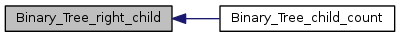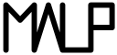Multi-ApplicationOnlineProfiling  2.1
Binary Tree

This is the binary tree topology used by MALP for its internal communications. More...

## Data Structures

struct  Binary_Tree
Struct defining a binary tree. More...

## Functions

void Binary_Tree_init (struct Binary_Tree *bt, int rank, int size)
Initialization of a binary tree. More...

void Binary_Tree_release (struct Binary_Tree *bt)
releases a binary tree More...

static int Binary_Tree_parent (struct Binary_Tree *bt)
Getter on the parent. More...

static int Binary_Tree_left_child (struct Binary_Tree *bt)
Getter on the left child. More...

static int Binary_Tree_right_child (struct Binary_Tree *bt)
Getter on the right child. More...

static int Binary_Tree_child_count (struct Binary_Tree *bt)

## Detailed Description

This is the binary tree topology used by MALP for its internal communications.

This is used to build a mpi process topology for communication. It is basically used in the reduction process (see Red_Ht) Each mpi process creates a Binary_Tree with their rank (given by MPI_Comm_rank) and run size (given by MPI_Comm_size). For a given size and rank, parent and children can be found with initializing a Binary_Tree with Binary_Tree_init.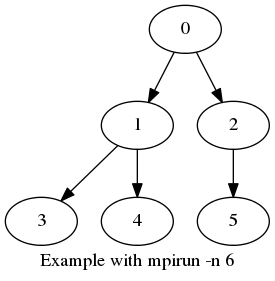## Function Documentation

 static int Binary_Tree_child_count ( struct Binary_Tree * bt )
inlinestatic

Definition at line 90 of file Binary_Tree.h.

Here is the call graph for this function: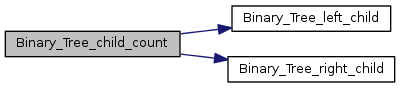void Binary_Tree_init ( struct Binary_Tree * bt, int rank, int size )

Initialization of a binary tree.

Parameters
 bt the tree to initialize rank the rank of initialized tree size the size of the tree

Definition at line 22 of file Binary_Tree.c.

 static int Binary_Tree_left_child ( struct Binary_Tree * bt )
inlinestatic

Getter on the left child.

Parameters
 bt the Binary_Tree
Returns
the left_child of given Binary_Tree

Definition at line 75 of file Binary_Tree.h.

Here is the caller graph for this function: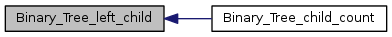static int Binary_Tree_parent ( struct Binary_Tree * bt )
inlinestatic

Getter on the parent.

Parameters
 bt the Binary_Tree
Returns
the parent of given Binary_Tree

Definition at line 65 of file Binary_Tree.h.

 void Binary_Tree_release ( struct Binary_Tree * bt )

releases a binary tree

Parameters
 bt the binary tree to release

Definition at line 67 of file Binary_Tree.c.

 static int Binary_Tree_right_child ( struct Binary_Tree * bt )
inlinestatic

Getter on the right child.

Parameters
 bt the Binary_Tree
Returns
the right_child of given Binary_Tree

Definition at line 85 of file Binary_Tree.h.

Here is the caller graph for this function: